Home > A2C > Chapter 2 > Lesson 2.2.2 > Problem2-137

2-137.
1. Jenna wants to solve the equation 2000x − 4000 = 8000. Homework Help ✎

1. What easier equation could she solve instead that would give her the same solution? (In other words, what equivalent equation has easier numbers to work with?)

2. Justify that your equation in part (a) is equivalent to 2000x − 4000 = 8000 by showing that they have the same solution.

3. Now Jenna wants to solve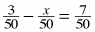. Write and solve an equivalent equation with easier numbers that would give her the same answer.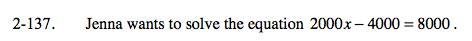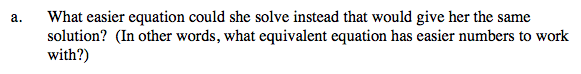Divide both sides by 2000.

x − 2 = 4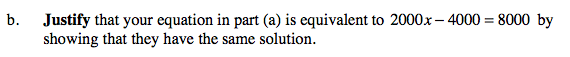Solve both equations.

2000x − 4000 = 8000

2000x = 12000

x − 2 = 4

x = 6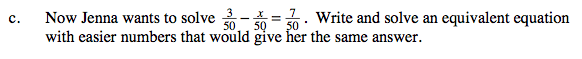Multiply both sides by 50 and simplify. Remember to check your work.